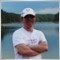# difference in moving averages.[Deleted]
Hello,
ive bin really, really trying to code an EA function that would do the following.

It would find the value of the MA in the last two closed bars and see if their is a difference of 'MinAng' in are case 5, between the two and in what direction.

ex.
shift 1 - 2.0325 (one bar back)
shift 2 - 2.0321 ( two bar back)

25
-21
____
4 4 is not equal or greater than 5 so return 0

I've tryed the following but it only works some of the time and only when the difference is greater than 5... not equal.

extern int angle = 5;
`int Mangle = MAngle(angle);`

```int MAngle(double MinAng)
{
double thspos = NormalizeDouble(iMA (NULL, 0, period, 0, MODE_SMA, PRICE_CLOSE, 1), Digits);
double lstpos = NormalizeDouble(iMA (NULL, 0, period, 0, MODE_SMA, PRICE_CLOSE, 2), Digits);

if (thspos >= (lstpos + MinAng*Point)) {return(1);} //up
if (thspos <= (lstpos - MinAng*Point)) {return(2);} //down
return(0);
}```
the rest of the code that involves this function is with the buy/sell. but that only uses the what it returns (1, 2, 0).Admin
17368

See Odd comparing two doubles
int x = double y ?

Comparsion Problem[Deleted]
Rosh:
See Odd comparing two doubles
int x = double y ?

Comparsion Problem

I looked into those threads and it seems that the solutions were to use the "NormalizeDouble" subroutine.
would it be better just to define my MAs as strings? i already used that subroutine and it still didn't work.2646

You didn't use it here:

if (thspos >= (lstpos + MinAng*Point)) {return(1);} //up
if (thspos <= (lstpos - MinAng*Point)) {return(2);} //down[Deleted]
yup that did the trick phy.

it seems it didnt carry over from when i declared them.